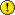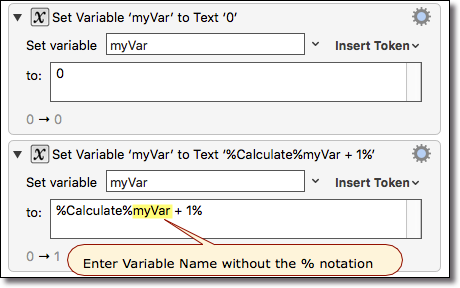#Keyboard Maestro Wiki

token:Calculate

## Calculate Token

The %Calculate%<formula>% token returns the numeric result of performing a calculation, where `<formula>` may be any of the following:

• Mathematical formula like `(2 + 3)*12`
• Use any Variable which may be evaluated as a number
•Just enter the Variable Name (without the `%`) in the Calculate token `<formula>`
• Examples:
• `%Calculate%MyVar + 1%`
• `%Calculate%(MyVarWordsPerSec * 60)/MyVarWordsPerPage%`
• Use any of the built-in Keyboard Maestro functions, like RANDOM(10)
• Get the numeric value of an Variable array element, like `MyVar`, if MyVar is `10,05,12,08` returns `5` (Arrays may contain only numeric data)

The %Hex2% token (v8+) returns the value in Hex format, padded with zeros to two digits. “2” is just an example, you can use %Hex8% for example.

The %Dec2% token (v8+) returns the value in decimal format, padded with zeros to two digits (similarly %Dec5% to five digits, etc).

The %Oct3% token (v8+) returns the value in octal format, padded with zeros to three digits (similarly % Oct5% to five digits, etc).

The %Bin8% token (v8+) returns the value in binary format, padded with zeros to eight digits (similarly % Bin16% to 16 digits digits, etc).

Example ActionA reminder that the following are only equivalent if the Variable is numeric:

• %Variable%Count% (Variable)
• %Calculate%Count% (Number)

So, if MyVar is “`005`”, then

• %Variable%MyVar% will return `005`
• %Calculate%MyVar% will return `5`